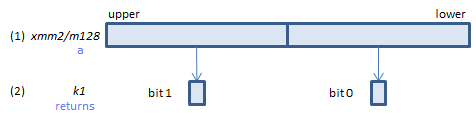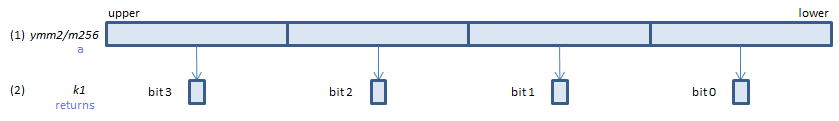﻿ vfpclasspd

## VFPCLASSPD - Floating-Point CLASS Packed Double

VFPCLASSPD k1{k2}, xmm2/m128/m64bcst, imm8    (V5+DQ+VLFor each double, if (1) belongs to one of the classes specified in imm8, set 1, else set 0, to the corresponding bit of (2).
If k2 bit is 0, the corresponding bit of (2) is cleared. Upper bits of (2) are zeroed.
VFPCLASSPD k1{k2}, ymm2/m256/m64bcst, imm8    (V5+DQ+VLFor each double, if (1) belongs to one of the classes specified in imm8, set 1, else set 0, to the corresponding bit of (2).
If k2 bit is 0, the corresponding bit of (2) is cleared. Upper bits of (2) are zeroed.
VFPCLASSPD k1{k2}, zmm2/m512/m64bcst, imm8    (V5+DQ